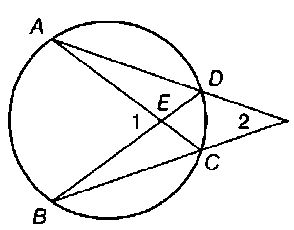Chapter 6.CT, Problem 5CTElementary Geometry For College St...

7th Edition
Alexander + 2 others
ISBN: 9781337614085

Solutions

Chapter
SectionElementary Geometry For College St...

7th Edition
Alexander + 2 others
ISBN: 9781337614085
Textbook Problem

Given that m A B ⌢ = 106 ° and m D C ⌢ = 32 ° , find:a) m ∠ 1 b) m ∠ 2To determine

(a)

To find:

m1.

Explanation

Theorem used:

The measure of an angle formed by two chords that intersect within a circle is one-half the sum of the measures of the arcs intercepted by the angle and its vertical angle.

Calculation:

Given:

mAB=106° and mDC=32°.

Consider the given figure,

From the theorem,

To determine

(b)

To find:

m2.

Still sussing out bartleby?

Check out a sample textbook solution.

See a sample solution

The Solution to Your Study Problems

Bartleby provides explanations to thousands of textbook problems written by our experts, many with advanced degrees!

Get Started

In problems 17-30, simplify each expression so that only positive exponents remain. 19.

Mathematical Applications for the Management, Life, and Social Sciences

Show that limn(1+xn)n=exfor any x 0.

Single Variable Calculus: Early Transcendentals, Volume I

Proof prove that abx2dx=b3a33.

Calculus: Early Transcendental Functions

Solve the equations in Exercises 126. x1x=0

Finite Mathematics and Applied Calculus (MindTap Course List)

Evaluate the integral. 2. sin3cos4d

Single Variable Calculus: Early Transcendentals